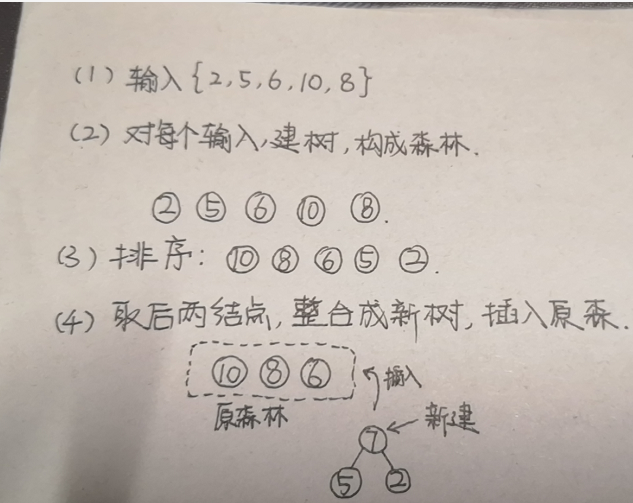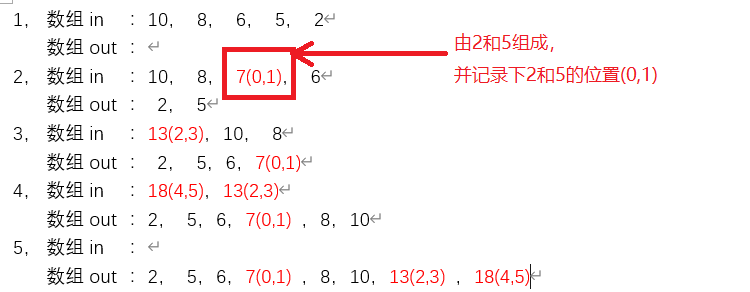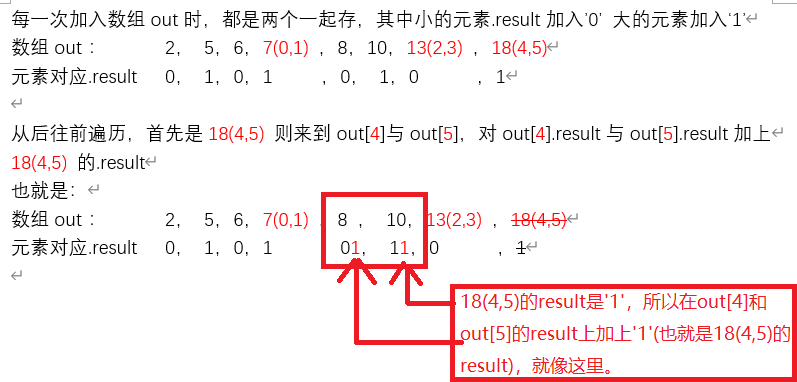# (数据结构与算法)哈夫曼编码计算方法(两种实现方法)

### 哈夫曼编码

①将输入的数值，每一个数值，分别都建立成一棵树(只有根节点的树)，就形成了森林。
②将森林排序( 按权重，从大到小排序，选用插入排序即可，因为之后的排序每一次都只插入一个数值，只需要移动少量即可)
③取出后两棵树n1、n2，新创建一个根节点，然后将n1、n2作为其左右孩子，从而构成一棵新树(由新建根节点+n1(lchild)+n2(rchild)组成)，将这棵新树插入森林中。
④重复②③，直到森林中只剩下一棵树，此时该树就是所求哈夫曼树。
⑤遍历每一个叶子(每一个叶子就是输入数值)，根据遍历路径(往lchild则 +‘1’，往rchild 则 + ‘0’)，每一个叶子对应的数值+编码result，即是其哈夫曼编码。#include <bits/stdc++.h>
using namespace std;
typedef struct tree_node{
int temp;				//存数值/对应字符
int weight;				//存权重
tree_node* lchild;
tree_node* rchild;
vector<int> result;		//存编码结果
tree_node(int t = -1, int w = 0) {	//非叶子结点，temp都等于-1
temp = t;
weight = w;
lchild = NULL;
rchild = NULL;
}
};
class huffma{
public:
void input(int* data, int len) {	//一开始是选用vector<int>，但是朋友说输入是需要数组，所以也就在这里转一下vector<int> 哈哈哈
vector<int>data_in(data, data + len);
make_trees(data_in);		//建树
}
void output() {	//输出
vector<int> a;
make_result(tree, a, 0);	//找编码
print_result(tree);			//输出编码
}
private:
tree_node* tree = new tree_node;		//做根结点
vector<tree_node*> trees;		//森林
void sort_trees() {		//森林排序：插入排序
for (int i = 1; i < trees.size(); i++) {
tree_node* change = trees[i];
int j = i - 1;
while (change->weight > trees[j]->weight) {
trees[j + 1] = trees[j];
j--;
if (j == -1)break;
}
trees[j + 1] = change;
}
}
void built_it() {			//建树
int len = trees.size();
sort_trees();
while (len >= 3) {
tree_node* n1 = trees.back();
trees.pop_back();
tree_node* n2 = trees.back();
trees.pop_back();
tree_node* new_node = new tree_node(-1, n1->weight + n2->weight);//temp = -1，表示非叶子结点
new_node->lchild = n1;
new_node->rchild = n2;
trees.push_back(new_node);
sort_trees();
len = trees.size();
}		//剩下最后两棵，直接作为创建的根节点的左右孩子
tree_node* n1 = trees.back();
trees.pop_back();
tree_node* n2 = trees.back();
trees.pop_back();
tree->lchild = n1;
tree->rchild = n2;
}
void make_result(tree_node* p, vector<int> a, int add) {		//递归，找出编码
for (int i = 0; i < a.size(); i++)p->result.push_back(a[i]);
if (p->lchild != NULL)make_result(p->lchild, p->result, 0);
if (p->rchild != NULL)make_result(p->rchild, p->result, 1);
}
void print_result(tree_node* p) {			//打印出叶子结点的result
if (p->temp != -1) {
cout << p->temp << "->";
for(int i = 1;i<p->result.size();i++) cout << p->result[i];
cout << endl;
}
if (p->lchild != NULL)print_result(p->lchild);
if (p->rchild != NULL)print_result(p->rchild);
}
void make_trees(vector<int> data) {		//vector<int>存入森林，并built_it()
for (int i = 0; i < data.size(); i++) {
tree_node* new_tree = new tree_node(data[i], data[i]);
trees.push_back(new_tree);
}
built_it();
}
};
int main() {
huffma s;
int data = {2,5,6,8,13,19,25,36};		//输入：元素表示：字符和权重(相同)
int len = sizeof(data) / sizeof(data);
s.input(data,len);
s.output();
return 0;
}


①将输入数组存入 数组in
②对 数组in 进行排序(从大到小，这样在后两位、插入时都不需要移太多位)
③取出 数组in 的后两位，分别对其.result 赋值0或1，存到 数组out中，新建一个元素(=两元素的权值合，并记录子元素的位置，也即由两位合成后，记录这两位在 数组out 中的位置)，将新建的元素插入 数组in。
④重复②③，知道 数组in 为空。
⑤此时由后往前遍历 数组out，对每一位元素进行操作：根据该元素的记录位置，找到 数组out 中对应的元素，添加上该元素的赋值。(按照下图来说明比较容易)/*

*/

#include <bits/stdc++.h>
using namespace std;
typedef struct tree_node {
int temp;		//存元素：字符
int weight;		//权重
vector<int> result;		//存其result
vector<int> flag;		//存其两个合成的子元素的out数组位置
tree_node(int temp = -1, int weight = 0) {		//新建元素皆temp = -1
this->temp = temp;
this->weight = weight;
}
};
void node_sort(vector<tree_node>& data_in) {		//插入排序
for (int i = 1; i < data_in.size(); i++) {
tree_node change = data_in[i];
int j = i - 1;
while (change.weight > data_in[j].weight) {
data_in[j + 1] = data_in[j];
j--;
if (j == -1)break;
}
data_in[j + 1] = change;
}
}
void get_result(vector<tree_node>& data_out,int ing) {		//根据flag，去写对应out[]的result
if(data_out[ing].flag.size() > 0){
for(int i = 0;i< data_out[ing].result.size();i++){
data_out[data_out[ing].flag].result.push_back(data_out[ing].result[i]);
data_out[data_out[ing].flag].result.push_back(data_out[ing].result[i]);
}
}
}
void opperate(int* data, int len) {				//整个过程
vector<tree_node>data_in;		//数组in
vector<tree_node>data_out;		//数组out
for (int i = 0; i < len; i++) {		//读入输入
tree_node node = tree_node(data[i], data[i]);
data_in.push_back(node);
}
node_sort(data_in);			//排序
int k = 0;
while (data_in.size() > 1) {		//重复②，③
tree_node n1 = data_in.back();
data_in.pop_back();
n1.result.push_back(0);		//较小元素，则添加result    '0'
data_out.push_back(n1);
tree_node n2 = data_in.back();
data_in.pop_back();
n2.result.push_back(1);		//较大元素，则添加result    '1'
data_out.push_back(n2);
tree_node n3 = tree_node(-1, n1.weight + n2.weight);
n3.flag.push_back(k++);
n3.flag.push_back(k++);
data_in.push_back(n3);
node_sort(data_in);
}
//从后往前遍历
for (int i = data_out.size()-1; i >= 0; i--)get_result(data_out, i);
//依次输出out数组里(temp !=1 的元素)
for (int i = 0; i < data_out.size(); i++) {
if (data_out[i].temp != -1) {
cout << data_out[i].temp << "->";
for (int j = data_out[i].result.size() - 1; j >= 0; j--)cout << data_out[i].result[j];
cout << endl;
}
}
}
int main() {
int data = { 2,5,6,8,13,19,25,36 }; 		//输入：元素表示：字符和权重(相同)
int len = sizeof(data) / sizeof(data);
opperate(data, len);
return 0;
}（左为方法一，右为方法二。结果一致，但方法二的结果是从权重低的开始排起，所以在使用中，“对比”、“查找”等操作则会方便很多,从时间上来说，两个方法差别不大，测试了128个字符时，并没有多大的时间差别)

①选用插入排序，主要是考虑到后续插入时只插入一个，都是O(n)，应该时间消耗不是很大。可能选用其他排序更优，但是有一点值得提的是，插入排序是稳定排序(稳定排序，当新建结点 与 输入元素 权重相同时，可以优先取新建结点，这样会使较深层叶子结点用少一丢丢的编码长度，当然我只是脑补一下这个结果，真实情况再来探讨，所以先保留为待优化空间)
②在修改下一层的result时，总是用
for(int i = 0;i< data_in.size();i++)data.push_back( data_in[i])，这里重复度很高。这里有很大的时间浪费在这里。所以这里有很大的优化空间。这里占用时间高达50%以上（粗略测试，该数值仅供参考）有一个新的想法是：重新遍历一遍，再依次写下。理论上可能会更快，闲时再来试一试。

09-20750904-04262
12-172880
11-294万+
12-211万+
09-112452
02-024759
07-1558
12-182万+
10-13536